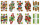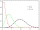# Binomial distribution + reason - math problems

#### Number of problems found: 13

• Error rateThe exam has 6 questions. Students have an error rate of 20% and can have a maximum of 1 question wrong. What is the probability that they will succeed?
• Poisson distribution - daisiesThe meadow behind FLD was divided into 100 equally large parts. Subsequently, it was found that there were no daisies in ten of these parts. Estimate the total number of daisies in the meadow. Assume that daisies are randomly distributed in the meadow.
• SeedsFrom a box of spruce seeds with the germination of 80%, we randomly select 10 seeds and plant them. Find the median of the random variable: the number of germinating seeds.
• Playing cardsFrom 32 playing cards containing 8 red cards, we choose 4 cards. What is the probability that just 2 will be red?
• Six questions testThere are six questions in the test. There are 3 answers to each - only one is correct. In order for a student to take the exam, at least four questions must be answered correctly. Alan didn't learn at all, so he circled the answers only by guessing. What
• The testThe test contains four questions, and there are five different answers to each of them, of which only one is correct, the others are incorrect. What is the probability that a student who does not know the answer to any question will guess the right answer
• RecordsRecords indicate 90% error-free. If 8 records are randomly selected, what is the probability that at least 2 records have no errors?
• Boys and girlsThere are 11 boys and 18 girls in the classroom. Three pupils will answer. What is the probability that two boys will be among them?
• Left handedIt is known that 25% of the population is left-handed. What is the probability that there is a maximum of three left-handers at a seminar where there are 30 participants?
• DiceWe throw five times the dice. What is the probability that six fits exactly twice?
• TestThe teacher prepared a test with ten questions. The student can choose one correct answer from the four (A, B, C, D). The student did not get a written exam at all. What is the probability that: a) He answers half correctly. b) He answers all correctly. c
• TV failThe TV has after 10,000 hours average 35 failures. Determine the probability of TV failure after 400 hours of operation.
• FamilyWhat is the probability that a family with 7 childrens have: exactly 6 dívek? 2 dívky and 5 boys? Consider the birth probability of a girl is 48.87% and boy 51.13%.

We apologize, but in this category are not a lot of examples.
Do you have an exciting math question or word problem that you can't solve? Ask a question or post a math problem, and we can try to solve it.

We will send a solution to your e-mail address. Solved examples are also published here. Please enter the e-mail correctly and check whether you don't have a full mailbox.

Binomial distribution - math problems. Reason - math problems.